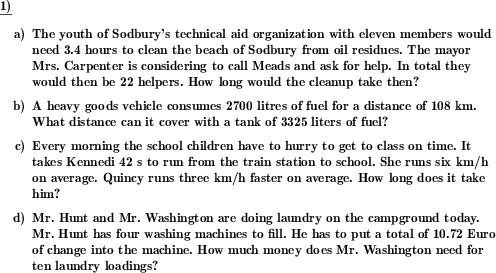Custom math worksheets at your fingertips# Details for problem "Rule of three word problem"

Quickname: 3894

Elementary School, Primary School, Junior High School, Middle School, High School.

## Summary

Word problems that can be solved by applying the rule of three.

## Example## Description

Simple word problems with direct or indirect proportional relations.

The maximum size of the proportionality constant can be set. For tasks with money this relates to the number of cents of an amount, for tasks involving time this is the maximum number of seconds.

The maximum size of the initial divisor (by which to divide to obtain the proportionality constant), the target multiplier (by which to multiply to obtain the value sought) can also be limited.

Since the conversion from cents to euros may present an additional challenge, it can be determined whether such tasks are allowed.

The number of problems can also be selected.

Download free printable worksheets for this math problem here. The worksheet contains the problems only, the solution sheet includes the answers. Just click on the respective link.

•Worksheet 1Solution sheet with answers
•Worksheet 2Solution sheet with answers
•Worksheet 3Solution sheet with answers

If you can not see the solution sheets for download, they may be filtered out by an ad blocker that you may have installed. If this is the case, please allow ads for this page and reload the page. The solution sheets will then reappear.

• Do these sample worksheets do not really fit?
• Do you need more math worksheets, with a different level of difficulty?
• Would you like to combine different problems on a worksheet and adjust them to your needs?
• As a teacher, you can put together your own worksheets using the automatically generated math problems provided.
With a free initial credit, you can start creating your own math worksheets in a few minutes.

You can try it for free! Register here, to create custom worksheets now!

## Customization options for this problem

Parameter
Possible values
Number of problems
1, 2, 3, 4
Whole numbers only
Yes, No
Unit conversion
Never, May, Always
Type of relation
Direct & inverse, Direct only, Inverse only
Difficulty level
Number as absolute value, Number can be increment, Number absolute/increment

## Similar problems

Remark
Description
In the form of a table: direct rule of three
Apply the rule of three for a direct proportionality relationship and fill in missing values in a table.
In the form of a table: indirect rule of three
Apply the rule of three for an inverse proportionality relationship and fill in missing values in a table.
Without the initial division, with direct specification of the proportionality constant
Easy word problems that can be solved by applying the rule of three. No initial division necessary, first factor is one.